# How to use trigonometric functions in Excel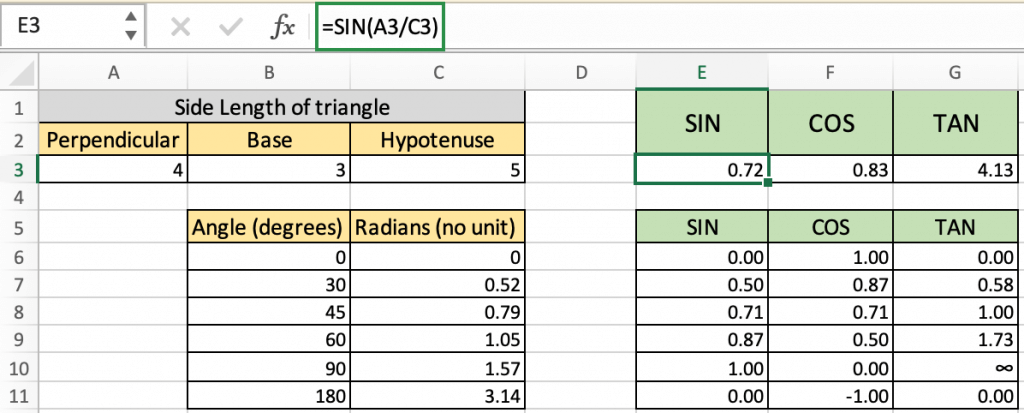In this article, we will learn How to use trigonometric functions in Excel.

What is trigonometry?

Trigonometry is a branch of mathematics that studies the relations between the elements (sides and angles) of a triangle. You might now be remembering many trigonometric formulas and equations you learned during your school or college days. Some of them are cot x = 1/tanx , six x/cos x = tan x, sin(900-x) - cos x and so on. Excel offers a number of built-in functions that deal with trigonometry. You can use these trig functions to solve complex trigonometric expressions.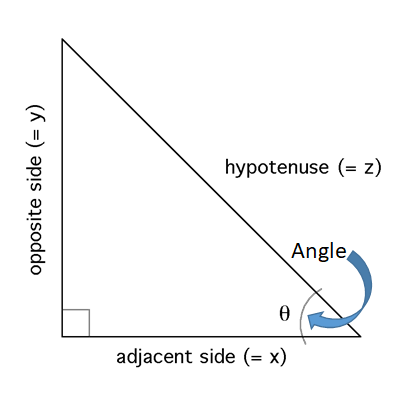The main thing you need to consider while solving trigonometric expressions is that Excel performs the calculations considering angle value in radians and not in degrees. You might know that sin 900 = 1 .So, if you enter the formula SIN (90) in Excel, the result will be .893997 and not 1 because Excel considers 90 as 90 radians and not 90 degrees. If you want to find the sine of 90 degrees, then you should first convert degrees into radians and then use the SIN formula available in Excel. Don’t worry, we are going to look at how to use trigonometric functions in Excel in minutes.

Excel provides functions for sine (sin), cosine (cos), tangent (tan), hyperbolic sine (sinh), hyperbolic cosine (cosh) and hyperbolic tangent (tanh). Excel does not provide functions for secant (sec), cosecant (cosec), cotangent (cot) and for their hyperbolic counterparts. However, you can calculate these functions using the core functions (sine and cosine). Excel also offers functions to convert angle from radians to degrees and vice versa.

Trigonometric functions

• SIN (Sine function)
• COS (cosine function)
• TAN (tan function)

Inverse Trigonometric functions

• ASIN (Inverse Sine function)
• ACOS (Inverse Cosine function)
• ATAN (Inverse Tan function)

Hyperbolic trigonometric functions

• SINH (Hyperbolic Sine function)
• COSH (Hyperbolic Cosine function)
• TANH (Hyperbolic Tan function)

When solving trigonometric expressions like sine, cosine and tangent, it is very important to realize that Excel uses radians, not degrees to perform these calculations! If the angle is in degrees you must first convert it to radians.

There are two easy ways to do this.

Recall that p = 180°. Therefore, if the angle is in degrees, multiply it by p/180° to convert it to radians. With Excel, this conversion can be written PI( )/180. For example, to convert 45° to radians, the Excel expression would be 45*PI( )/180 which equals 0.7854 radians. Excel has a built-in function known as RADIANS(angle) where angle is the angle in degrees you wish to convert to radians. For example, the Excel expression used to convert 270° to radians would be RADIANS(270) which equals 4.712389 radians

The RADIANS function takes value of angle in degrees and returns the angle in radians.

Syntax:

angle : angle in degrees

If using this formula =SIN(x/y) then we need not need to convert the value to radians. But if we are directly input the values

SIN function :

All of these might be confusing to understand. Let's understand how to use the function using an example. Here we have the length of the sides of the triangle.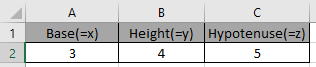These are the lengths of the Triangle having base, height and hypotenuse.

So we need to find the Sine of the Angle.

Use the formula:

 =SIN(A2/C2)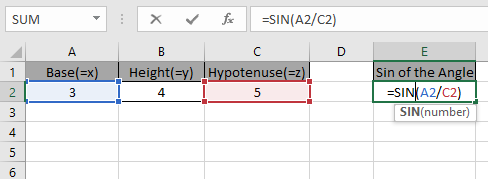Press Enter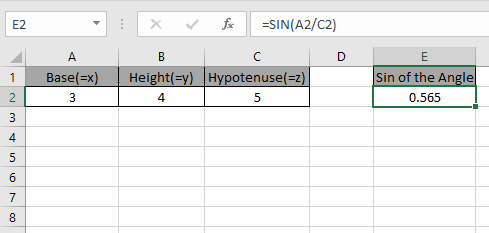As you can see the Sine of the angle of the triangle.

The above method was for having sides of the triangle. If you have the angle in degrees and you need to find the Sine of that angle.

Use the formula:

It will get you the Sine of the 30 degrees angle.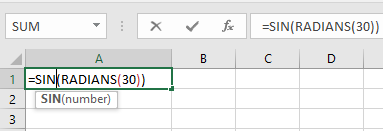Press Enter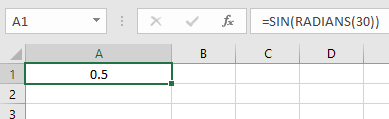Using PI()/180 method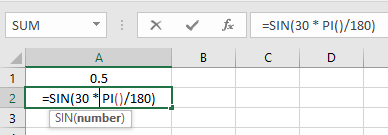Press Enter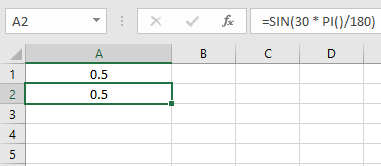As you can see the Sine of the angle using the SINE function.

COS function

Here we have the length of the sides of the triangle.These are the lengths of the Triangle having base, height and hypotenuse.

So we need to find the COS of the Angle.

Use the formula:

 =COS(B2/C2)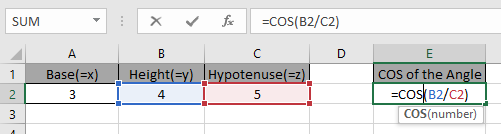Press Enter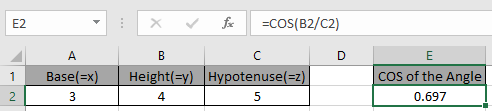As you can see the COS of the angle of the triangle.

The above method was for having sides of the triangle. If you have the angle in degrees and you need to find the COS of that angle.

Use the formula:

It will get you the COS of the 30 degrees angle.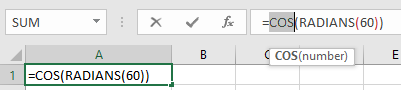Press Enter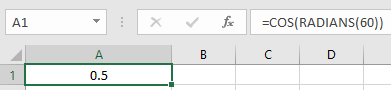Using PI()/180 method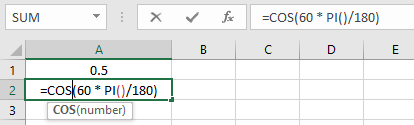Press Enter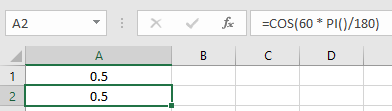As you can see the Cos of the angle using the COS function.

TAN function

HereThese are the length of sides of a Triangle having base, height and hypotenuse.

So we need to find the Tangent value of the Angle.

Use the formula:

 =TAN(A2/B2)

A2/B2 : Adjacent side of the angle is divided by the opposite side of the angle. This will return a number as an argument to the function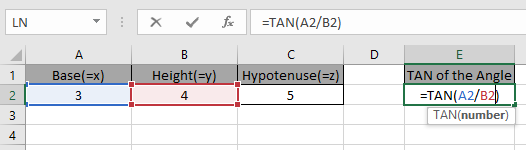Press Enter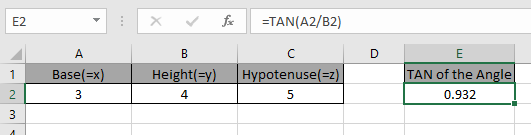As you can see the tangent of the angle of the triangle.

The above method was for having sides of the triangle. If you have an angle in degrees and you need to find the tangent of that angle.

Use the formula:

It will get you the Tan of the 30 degrees angle.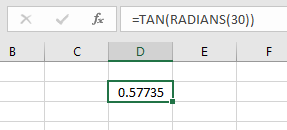Using PI()/180 method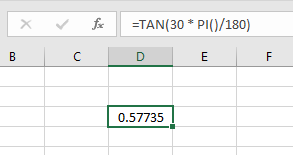As you can see the tangent of the angle using TAN function.

ASIN function

Here we have the length of the sides of the triangle.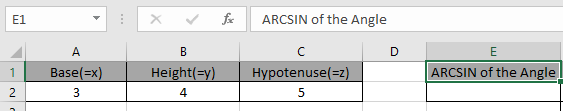Here we have the lengths of sides of the right - angle Triangle having sides as base, height and hypotenuse.

So we need to find the inverse Sine of the ratio of the sides.

Use the formula:

 =ASIN(A2/C2)

A2/C2 : it returns the ratio of the sides where value of the sides is given in as cell reference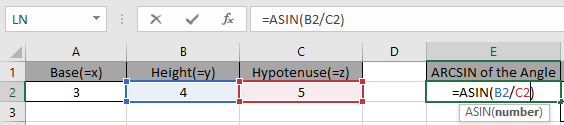Given the ratio of the sides as input to the ASIN function in excel and Press Enter.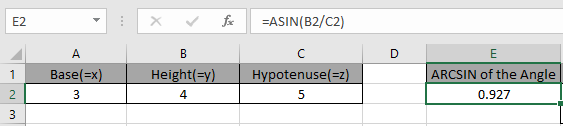As you can see, the ASIN function returns a value in radians.

The above method returns the value in radians. So to convert the value from radians to degrees use the DEGREES function or multiply the result with 180/PI().

Use the formula:

 = DEGREES ( ASIN ( E2 )

OR

 = SIN ( E2 ) * 180 / PI()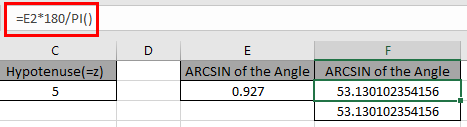The above snapshot gets the result as 53.13 degrees.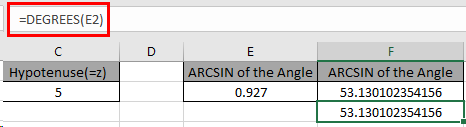The above snapshot uses the DEGREES function which takes the input in radians and returns the value in degrees.

As you can see, we can get the inverse of the Sine function using the ASIN function.

ACOS function

Here we have the length of the sides of the triangle.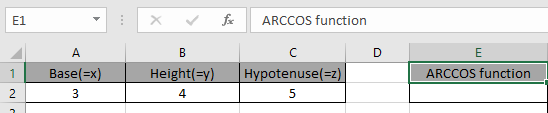Here we have the lengths of sides of the right - angle Triangle having sides as base, height and hypotenuse.

So we need to find the inverse Cosine of the ratio of the sides.

Use the formula:

 =ASIN(A2/C2)

A2/C2 : it returns the ratio of the sides where value of the sides is given in as cell reference.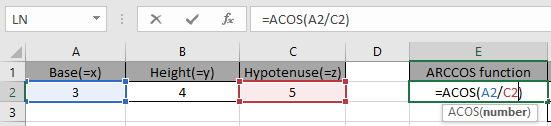Given the ratio of the sides as input to the ACOS function in excel and Press Enter.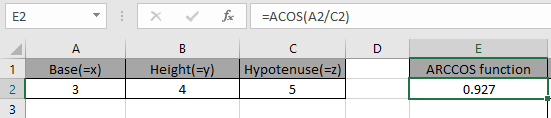As you can see, the ACOS function returns a value in radians.

The above method returns the value in radians. So to convert the value from radians to degrees use the DEGREES function or multiply the result with 180/PI().

Use the formula:

 = DEGREES ( ACOS ( E2 )

OR

 = COS ( E2 ) * 180 / PI()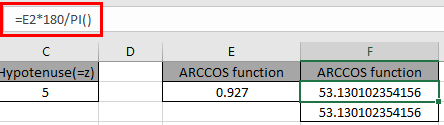The above snapshot gets the result as 53.13 degrees.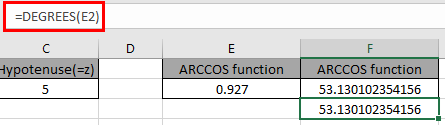The above snapshot uses the DEGREES function which takes the input in radians and returns the value in degrees.

ATAN function

Here we have the length of the sides of the triangle.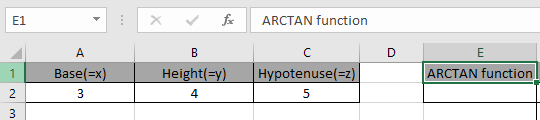Here we have the lengths of sides of the right - angle Triangle having sides as base, height and hypotenuse.

So we need to find the inverse tangent of the ratio of the sides.

Use the formula:

 =ATAN(A2/C2)

A2/C2 : it returns the ratio of the sides where value of the sides is given in as cell reference.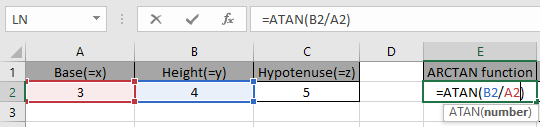Given the ratio of the sides as input to the ATAN function in excel and Press Enter.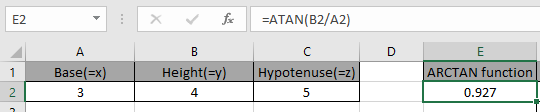As you can see, the ATAN function returns a value in radians.

The above method returns the value in radians. So to convert the value from radians to degrees use the DEGREES function or multiply the result with 180/PI().

Use the formula:

 = DEGREES ( ATAN ( E2 )

OR

 = TAN ( E2 ) * 180 / PI()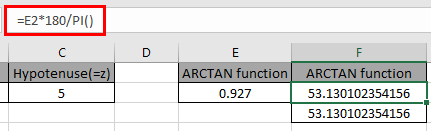The above snapshot gets the result as 53.13 degrees.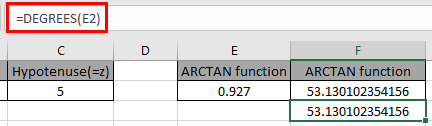The above snapshot uses the DEGREES function which takes the input in radians and returns the value in degrees.

Here are all the observational notes using the TAN formula in Excel
Note :

1. The TAN function returns an arbitrary value when an argument 90 degrees or (pi/2 = 1.5708…) is given but the actual value is infinity.
2. ASIN function returns in the range of -1.57.. ( -90 degrees ) to +1.57.. ( 90 degrees ).
3. ASIN function takes the input argument which should be between -1 to +1 or else the function returns the #NUM! Error.
4. The function returns #VALUE! Error if the argument to the function is non - numeric.

Hope this article about How to use trigonometric functions in Excel is explanatory. Find more articles on calculating values and related Excel formulas here. If you liked our blogs, share it with your friends on Facebook. And also you can follow us on Twitter and Facebook. We would love to hear from you, do let us know how we can improve, complement or innovate our work and make it better for you. Write to us at info@exceltip.com.

Related Articles :

How to use the SIN function in Excel : Sine value of an angle of a triangle using the SIN function in Excel.

How to use the COS function in Excel : Cosine value of an angle of a triangle using the SIN function in Excel.

How to use the TAN function in Excel : Tan value of an angle of a triangle using the SIN function in Excel.

How to use the SINH function in Excel : Sine hyperbolic value of an angle of a triangle using the SIN function in Excel.

How to use the COSH function in Excel : Cosine hyperbolic value of an angle of a triangle using the SIN function in Excel.

How to use the TANH function in Excel : Tan hyperbolic value of an angle of a triangle using the SIN function in Excel.

Popular Articles :